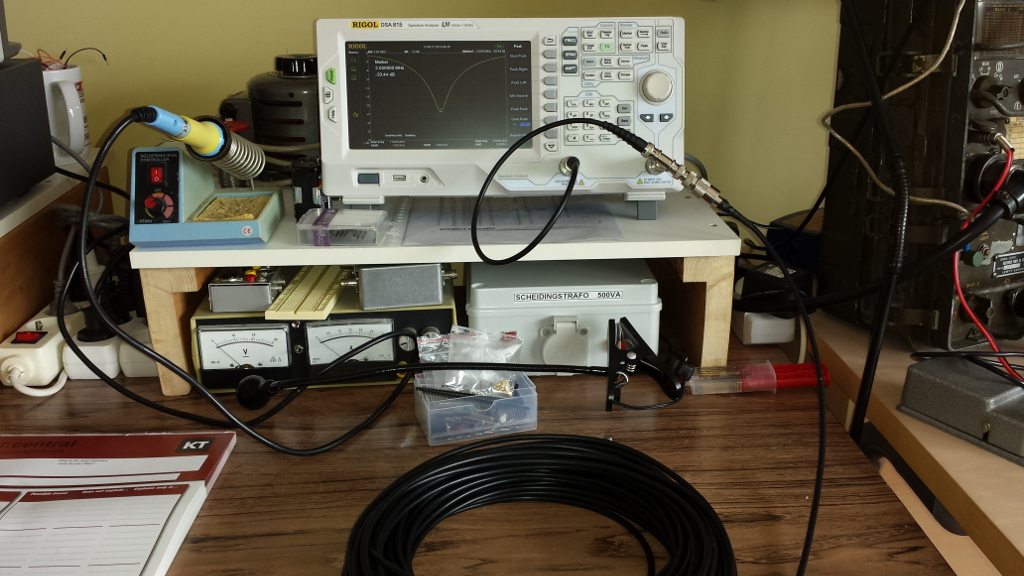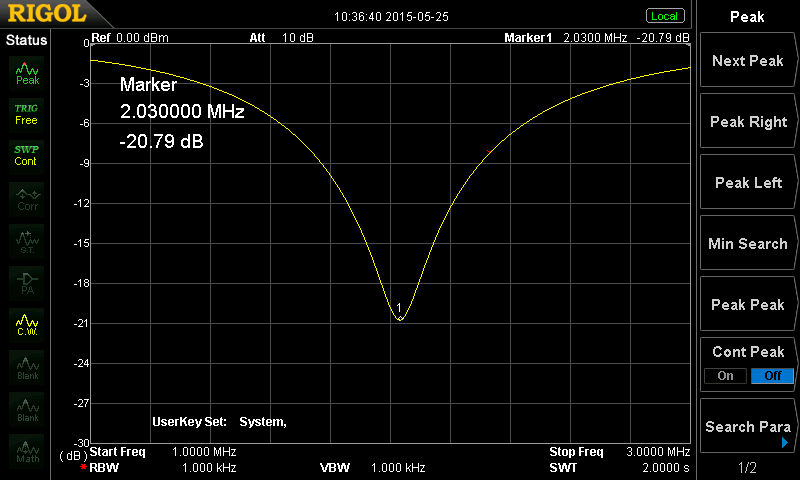# Measure coax length with a Spectrum analyser

### The measurement

The tracking generator of my Rigol DSA-815TG is connected to the Spectrum analyser input, with a T-connector, which also has the roll of coax (Velleman RG-58) connected. The setup is shown in the picture below.The picture taken from the Spectrum generator is:### The measurement

The dip in the measured trace is at 2.030MHz The known formula that relates wavelength (λ), lightspeed (c), velocity factor (v) and frequency (f) is:

 f = c * v λ

Where: c = 3 . 108 m/s and an estimation for the velocity factor found on the internet for my coax cable is 0.66.

The mechanism in use here, is that the other end of the roll of coax is left open (nothing connected to the connector). As a result all signal sent into the coax cable is reflected by its open end. This means that for signals with a frequency such that the cable length is a quarter wavelength, the reflected signal will pretty much completely be the inverse of the sent signal. As these two add up at the beginning of the cable, you would expect to see a strong dip for that frequency in the measurement setup above.

This strong dip is measured for 2.030MHz. The wavelength for this signal in this coax cable can be calculated as:

 λ = c * v = 3 . 108 * 0.66 =  97.54m f 2.03 . 106

As this is the full wavelength, this outcome is to be divided by 4 to get the length of the cable in use:

λ/4   =   97.54   /   4 =   24.38m

With this result I took the roll of coax and measured the real length using a tape measure. The length found was 23.42m.

So it can be concluded that the widely mentioned velocity factor of 0.66 for the coax in use here, can be used safely for future calculations.